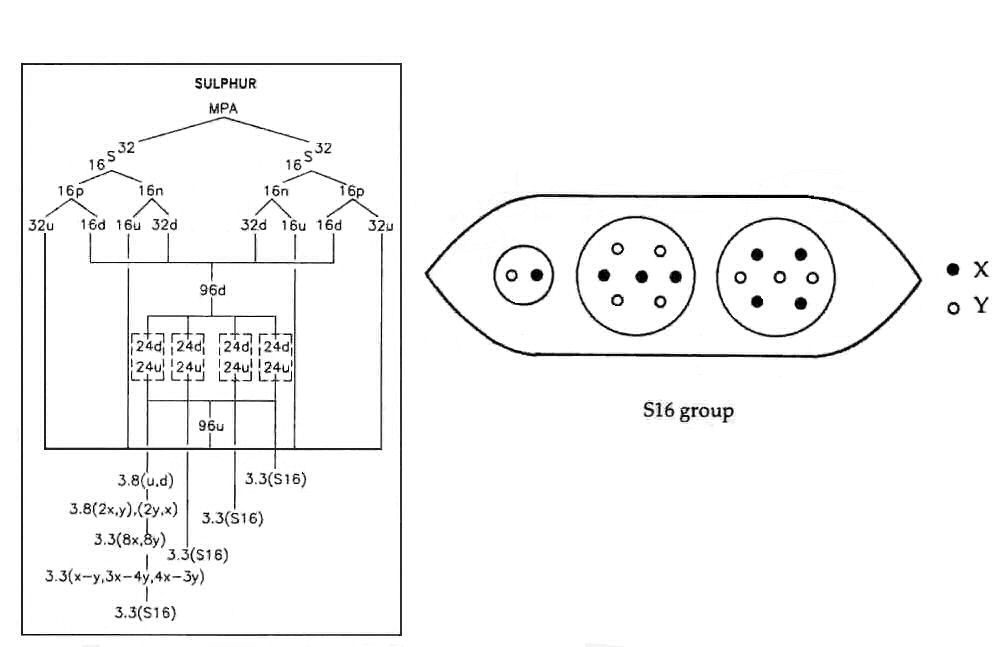The Sulphur MPAThe MPA is a tetrahedral array of four funnels. Each funnel has three segments. A segment contains three similar ovoids (S16). An ovoid encloses three small spheres, one containing a duad of UPAs (N2) and the other two containing groups of seven UPAs (I7).

Sulphur MPA = 4[3(3S16)].

The sulphur MPA is formed from two S32 nuclei, which provide 576 subquarks — the same as the number of UPAs. The ninety-six u quarks and ninety-six d quarks present in these nuclei are equally distributed among the four funnels. Each segment therefore contains the subquarks making up eight u quarks and eight d quarks. An S16 group consists of a X-Y bound state (N2), a 4X-3Y bound state (I7) and a 4Y-3X bound state (the mirror state I7′ of the former).The MPA of sulphur differs from that of magnesium in the replacement of an H3 triplet in the Mg12 group by an I7 group. An S32 nucleus contains four more protons and four more neutrons than a magnesium nucleus, that is, thirty-six more X subquarks and thirty-six more Y subquarks. The sulphur MPA has therefore seventy-two more X subquarks and thirty-two more Y subquarks than the magnesium MPA, that is, two more X and two more Y subquarks per ovoid. These additional four subquarks combine with the three subquarks in the u quark present in an ovoid of the magnesium MPA to form another I7:

Mg12 = N2 + I7′ + H3
= X-Y + 3X-4Y + u;

Mg12 + 2X + 2Y = X-Y + 3X-4Y + u (= 2X-Y) + 2X + 2Y
= X-Y + 3X-4Y + 4X-3Y

= N2 + I7′ + I7
= S16.

The disintegration diagram shows that the N2 is a (0) duad, i.e., an X-Y bound state. The bound state of seven UPAs depicted here is the mirror state I7′ (3X-4Y) of I7 because it breaks up into a quartet of UPAs and a (−) triplet (d quark):

3X-4Y → 2X-2Y + d quark (= X-2Y).

However, the other bound state of seven UPAs is an I7 (4X-3Y), which breaks up into a quartet of UPAs and a u quark:

4X-3Y → 2Y-2X + u quark (= 2X-Y).

These two bound states should break up into a (+) and a (−) triplet, not two (−) triplets, as the disintegration diagram indicates. As encountered in several previous analyses of MPAs, Besant & Leadbeater, presumably, did not examine both I7 groups to determine their positivity or negativity. Instead, they induced the break-up of only one group, assuming wrongly that, as both groups looked similar, their products of disintegration would be the same as well. That the difference in the predicted subquark contents of the nuclei of magnesium and sulphur should be precisely that needed to form an extra I7 group in each ovoid of the sulphur MPA is remarkable confirmation of the interpretation of MPAs as objects formed from two atomic nuclei. It is also convincing evidence that the predicted subquark composition of up and down quarks:

u = X-X-Y,      d = X-Y-Y

is correct.

Home